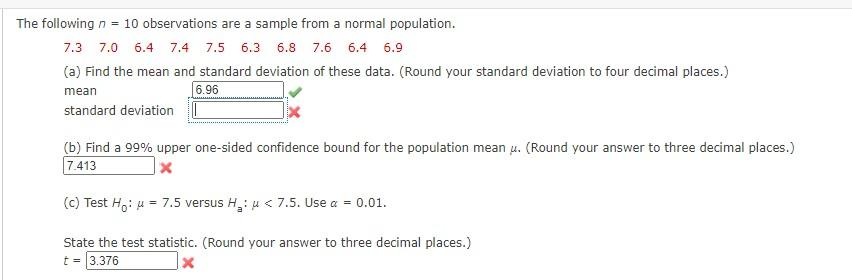# Question The following n = 10 observations are a sample from a normal population. 7.3 7.0 6.4 7.4 7.5 6.3 6.8 7.6 6.4 6.9 (a) Find the mean and standard deviation of these data. (Round your standard deviation to four decimal places.) mean 6.96 standard deviation (b) Find a 99% upper one-sided confidence bound for the population mean u. (Round your answer to three decimal places.) 7.413 X (c) Test Ho: u = 7.5 versus H, u < 7.5. Use a = 0.01. State the test statistic. (Round your answer to three decimal places.) t = 3.376G6W8JS The Asker · Probability and StatisticsTranscribed Image Text: The following n = 10 observations are a sample from a normal population. 7.3 7.0 6.4 7.4 7.5 6.3 6.8 7.6 6.4 6.9 (a) Find the mean and standard deviation of these data. (Round your standard deviation to four decimal places.) mean 6.96 standard deviation (b) Find a 99% upper one-sided confidence bound for the population mean u. (Round your answer to three decimal places.) 7.413 X (c) Test Ho: u = 7.5 versus H, u < 7.5. Use a = 0.01. State the test statistic. (Round your answer to three decimal places.) t = 3.376
More
Transcribed Image Text: The following n = 10 observations are a sample from a normal population. 7.3 7.0 6.4 7.4 7.5 6.3 6.8 7.6 6.4 6.9 (a) Find the mean and standard deviation of these data. (Round your standard deviation to four decimal places.) mean 6.96 standard deviation (b) Find a 99% upper one-sided confidence bound for the population mean u. (Round your answer to three decimal places.) 7.413 X (c) Test Ho: u = 7.5 versus H, u < 7.5. Use a = 0.01. State the test statistic. (Round your answer to three decimal places.) t = 3.376